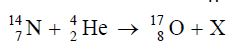Physic Tutorials

# How to solve Questions on Nuclear physics for UTME

How to solve Questions on Nuclear physics for UTME

Question 1

A piece of radioactive material contains 1000 atoms. If its half-life is 20 seconds, the time taken for 125
atoms to remain is {UTME 2012}
A. 20 seconds            B. 40 seconds         C. 60 seconds        D. 80 seconds

Solution

N = N0 exp(-ℷt)

ℷ = 0.693/T

ℷ = 0.693/20

125 = 1000 exp(-0.03465t)

125/1000 = exp(-0.03465t)

1000/125 = exp(0.03465t)

8 = exp(0.03465t)

0.03465t = In 8

0.03465t = 2.0794415

t = 2.0794415 /0.03465 = 60 seconds

C is the correct option

Question 2

A radioactive isotope has a decay constant of 10-5s-1. Calculate its half life. {UTME 2011}
A. 6.93 x 10-6s      B. 6.93 x 10-5s     C. 6.93 x 105s      D. 6.93 x 104s

Solution

T = 0.693/ℷ

T = 0.693 / 0.00001

T = 6.93 x 10^4 s

D is the correct option

Question 3

The radioisotope 235U92 decays by emitting two alpha particles, three beta particles and a gamma ray. What is the mass and atomic numbers of the resulting daughter element? {UTME 2010}
A. 91 and 227       B. 92 and 238      C. 227 and 91       D. 215 and 88.

Solution

You should note that in nuclear reaction, proton number and nucleon number must be conserved

235U92 – 2(4He2) + 3(0e-1) +ϒ + 227X91

227X91 means nucleon number(mass number) is 227 and proton number(atomic number) is 91.

C is the correct option

Question 4

A piece of radioactive material contains 10^20 atoms. If the half life of the material is 20 seconds, the number of
disintegrations in the first second is {UTME 2009}
A. 3.47 x 1018       B. 6.93 x 1020       C. 3.47 x 1020          D. 6.93 x 1018

Solution

N = N0 exp(-ℷt)

ℷ = 0.693/T

ℷ = 0.693/20

N = 10^20 exp(-1*0.03465)

N = 10^20/1.03536 = 9.658 x 10^19

the number of disintegrations in the first second is = N0 – N = 10^20 – 9.658 x 10^19

the number = 0.342 x 10^19 = 3.42 x 10^18

Question 5

If the decay constant of a radioactive substance is 0.231s-1, the half-life is {UTME 2009}
A. 3.00s      B. 0.12s       C. 0.33s     D. 1.50s

Solution

T = 0.693/ℷ

T = 0.693 / 0.23

T = 3.01 s

A is the correct option

Question 6In the equation above, the particle X is {UTME 2008}
A. a proton         B. a neutron      C. an α-particle       D. a β-particle

Solution

In nuclear reaction, proton number and nucleon number must be conserved

On the reactant side, the total number of proton = 7 + 2 = 9, the total nucleon number = 14 + 4 = 18

For the product side to be conserved, the nucleon number on X must be 1 and proton number must be 1

That is, 1X1 = proton

A is the correct option.

Question 7

A radioactive substance has a half-life of 20 days. What fraction of the original radioactive nuclei will remain after 80
days? {UTME 2007}
A. 1/16      B. 1/8        C. 1/4       D. 1/32

Solution

let the original value be N

N – N/2 …20 days

N/2 – N/4 … 40days

N/4 – N/8 ….60 days

N/8 – N/16 …. 80 days

after 80 days 1/16 0f the original radioactive nuclei will remain

A is the correct option

Question 8

The time it will take a certain radioactive material with a half-life of 50 days to reduce to 1/32 of its original
number is {UTME 2005}
A. 150 days       B. 200 days        C. 250 days         D. 300 days.

Solution

let the original value be N

N – N/2…50 days

N/2 – N/4 …100 days

N/4 – N/8 …150 days

N/8 – N/16 … 200 days

N/16 – N/32 … 250 days

It will take 250 days for a certain radioactive material with a half-life of 50 days to reduce to 1/32 of its original
number

C is the correct option

Question 9In the reaction above, X is {UTME 2005}
A. proton          B. neutron          C. electron             D. neutrino

Solution

In nuclear reaction, proton number and nucleon number must be conserved

On the reactant side, the total number of proton = 92 + 0 = 92, the total nucleon number = 235 + 1 = 236

On the product side, the total number of proton = 56 + 36 + A = 92, A = 0,  the total nucleon number = 144 + 90 + 2Z = 236, Z =(236-234)/2 = 2/2 = 1

For the product side to be conserved, the nucleon number on X is 1 and proton number is 0

1X0 = neutron

B is the correct option

Recommended: Short notes on Nuclear physics

Recommended: Question and answer on Nuclear for A level

### Bolarinwa Olajire

A lecturer, Educationist, PhD student at FUNAAB, and a Blogger.